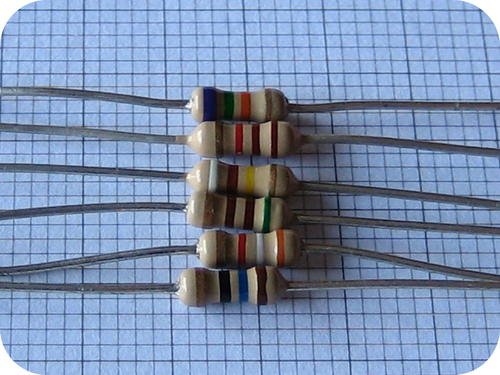# 1.5.1: Percent Error

$$\newcommand{\vecs}{\overset { \rightharpoonup} {\mathbf{#1}} }$$ $$\newcommand{\vecd}{\overset{-\!-\!\rightharpoonup}{\vphantom{a}\smash {#1}}}$$$$\newcommand{\id}{\mathrm{id}}$$ $$\newcommand{\Span}{\mathrm{span}}$$ $$\newcommand{\kernel}{\mathrm{null}\,}$$ $$\newcommand{\range}{\mathrm{range}\,}$$ $$\newcommand{\RealPart}{\mathrm{Re}}$$ $$\newcommand{\ImaginaryPart}{\mathrm{Im}}$$ $$\newcommand{\Argument}{\mathrm{Arg}}$$ $$\newcommand{\norm}{\| #1 \|}$$ $$\newcommand{\inner}{\langle #1, #2 \rangle}$$ $$\newcommand{\Span}{\mathrm{span}}$$ $$\newcommand{\id}{\mathrm{id}}$$ $$\newcommand{\Span}{\mathrm{span}}$$ $$\newcommand{\kernel}{\mathrm{null}\,}$$ $$\newcommand{\range}{\mathrm{range}\,}$$ $$\newcommand{\RealPart}{\mathrm{Re}}$$ $$\newcommand{\ImaginaryPart}{\mathrm{Im}}$$ $$\newcommand{\Argument}{\mathrm{Arg}}$$ $$\newcommand{\norm}{\| #1 \|}$$ $$\newcommand{\inner}{\langle #1, #2 \rangle}$$ $$\newcommand{\Span}{\mathrm{span}}$$Figure $$\PageIndex{1}$$ (Credit: Vald Kliper; Source: http://commons.wikimedia.org/wiki/File:Resistencias_250W_5%2525_sobre_papel_milimetrado.JPG; License: Public Domain)

### How does an electrical circuit work?

A complicated piece of electronic equipment may contain several resistors that have the role of controlling the voltage and current in the electrical circuit. If there is too much current, the apparatus malfunctions. Conversely, too little current means the system simply will not perform. The resistor values are always given with an error range. A resistor may have a stated value of 200 ohms, but a $$10\%$$ error range, meaning the resistance could be anywhere between 195 and 205 ohms. By knowing these values, an electronics person can design and service the equipment to make sure that it functions properly.

## Percent Error

An individual measurement may be accurate or inaccurate, depending on how close it is to the true value. Suppose that you are doing an experiment to determine the density of a sample of aluminum metal. The accepted value of a measurement is the true or correct value based on general agreement with a reliable reference. For aluminum, the accepted density is $$2.70 \: \text{g/cm}^3$$. The experimental value of a measurement is the value that is measured during the experiment. Suppose that in your experiment, you determine an experimental value for the aluminum density to be $$2.42 \: \text{g/cm}^3$$. The error of an experiment is the difference between the experimental and accepted values.

$\text{Error} = \text{experimental value} - \text{accepted value}\nonumber$

If the experimental value is less than the accepted value, the error is negative. If the experimental value is larger than the accepted value, the error is positive. Often, error is reported as the absolute value of the difference in order to avoid the confusion of a negative error. The percent error is the absolute value of the error, divided by the accepted value, and multiplied by $$100\%$$.

$\% \: \text{Error} = \frac{\left| \text{experimental value} - \text{accepted value} \right|}{\text{accepted value}} \times 100\%\nonumber$

To calculate the percent error for the aluminum density measurement, we can substitute the given values of $$2.45 \: \text{g/cm}^3$$ for the experimental value and $$2.70 \: \text{g/cm}^3$$ for the accepted value.

$\% \: \text{Error} = \frac{\left| 2.45 \: \text{g/cm}^3 - 2.70 \: \text{g/cm}^3 \right|}{2.70 \: \text{g/cm}^3} \times 100\% = 9.26\%\nonumber$

If the experimental value is equal to the accepted value, the percent error is equal to 0. As the accuracy of a measurement decreases, the percent error of that measurement rises.

## Summary

• Definitions of accepted value and experimental values are given.
• Calculations of error and percent error are demonstrated.

## Review

1. Define accepted value.
2. Define experimental value
3. What happens as the accuracy of the measurement decreases?

1.5.1: Percent Error is shared under a CC BY-NC license and was authored, remixed, and/or curated by LibreTexts.